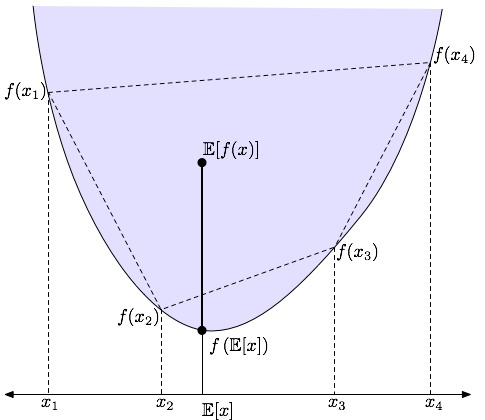# Jensen’s inequality – option pricing

This may also explain why a BM cubed isn’t a local martingale.

Q: How practical is JI?
A: practical for interviews.
A: JI is intuitive like ITM/OTM.
A: JI just says one thing is higher than another, without saying by how much, so it’s actually simpler and more useful than the precise math formulae. Wilmott calls JI “very simple mathematics”

JI is consistent with pricing math of vanilla call (or put). Define f(S) := (S-K)+. This hockey-stick is a kind of convex function. Now Under standard RN measure,

E[ f(S_T) ] should exceed f (E[ S_T ])

LHS is the call price today. RHS simplifies to f (S_0) := (S_0 – K)+ which is the intrinsic value today.

How about a binary call? Unfortunately, Not convex or concave !A graphical demonstration of Jensen’s Inequality. The expectations shown are with respect to an arbitrary discrete distribution over the xi